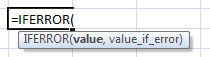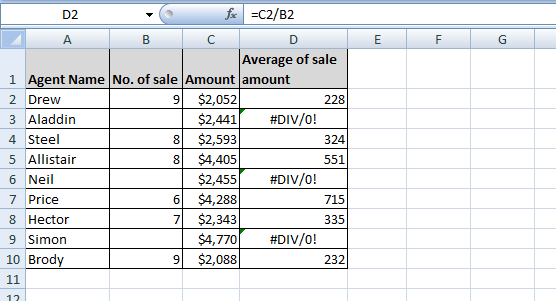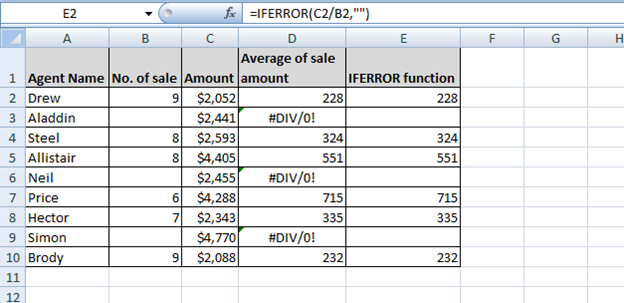# IFERROR Function - New Function in Excel 2007

In this article, we will learn where and how we can use Excel formula IFERROR.

What is IFERROR function?

The IFERROR function Returns a value you specify if a formula evaluates to an error;
otherwise, returns the result of the formula.Where do we use IFERROR function?

We use this function when we want to show value or any other text instead of error.

How do we use IFERROR function?

Let’s take an example and understand:-

We have agent’s sales data, in which we have details of Agent name, No. of sales, sales amount. For few agents, we do not have no. of sales so cells are blank and we want to return average sales amount for every agent.

In Excel, if we divide the one cell with the blank cell then formula returns #DIV/0! Error as result. As shown in below image:-Now we want that if we divide the sales amount with no. of sales then formula should return blank instead of #DIV/0!

To ignore #DIV/0! Error, we will use IFERROR function; follow below steps:-

• Enter the formula in cell E2.
• =IFERROR(C2/B2,"")
• Press Enter on your keyboard.
• Copy the same formula in the rest of the cells.
• The function will return only value including 0.Conclusion: - Whenever you want to ignore error while using the function and formulas in Excel, you can use this formula.